Courses

# NCERT Solutions(Part- 2)- Squares and Square Roots Class 8 Notes | EduRev

## Class 8 Mathematics by VP Classes

Created by: Full Circle

## Class 8 : NCERT Solutions(Part- 2)- Squares and Square Roots Class 8 Notes | EduRev

The document NCERT Solutions(Part- 2)- Squares and Square Roots Class 8 Notes | EduRev is a part of the Class 8 Course Class 8 Mathematics by VP Classes.
All you need of Class 8 at this link: Class 8

Question 1. Express the following as the sum of two consecutive integers.

(i) 212 (ii) 132 (iii) 112 (iv) 192

Solution:

(i) n = 21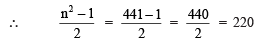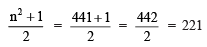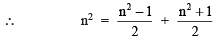or  212 = 220 + 221 = 441

(ii) n = 13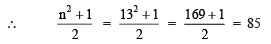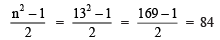∴ 132 = 85 + 84 = 169

Similarly,

(iii)112 = 60 + 61 = 121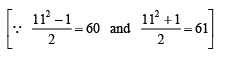(iv) 19= 180 + 181 = 361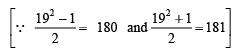Question 2. Do you think the reverse is also true, i.e. is the sum of any two consecutive positive integers is perfect square of a number? Give example to support your answer.

Solution: No, it is not always true.

For example:

(i) 5 + 6 = 11, 11 is not a perfect square.

(ii) 21 + 22 = 43, 43 is not a perfect square.

Property 10. The difference between the squares of two consecutive natural numbers is equal to the sum of the two numbers.

Examples:

92 – 82 = 81 – 64 = 17 = 9 + 8

102 – 92 = 100 – 81 = 19 = 10 + 9

15– 142 = 225 – 196 = 29 = 15 + 14

1012 – 1002 = 10201 – 10000 = 201 = 101 + 100

Property 11. If (n + 1) and (n – 1) are two consecutive even or odd natural numbers, then (n + 1) X  (n – 1) = n2 – 1.

For example,

10 * 12 = (11 – 1) * (11 + 1) = 112 – 1

11 * 13 = (12 – 1) * (12 + 1) = 122 – 1

25 * 27 = (26 – 1) * (26 + 1) = 262 – 1

Question 1: Write the square, making use of the above pattern.

(i) 1111112 (ii) 11111112

Solution: Using above pattern we can write,

(i) (111111)2 = 12345654321

(ii) (1111111)2 = 1234567654321

Question 2: Can you find the square of the following numbers using the above pattern?

(i) 66666672 (ii) 666666672

Solution: Using the above pattern, we can have,

(i) 6666667= 44444448888889

(ii) 666666672 = 4444444488888889

Exercise 6.1

Question 1. What will be the unit digit of the squares of the following numbers?

(i) 81 (ii) 272 (iii) 799 (iv) 3853 (v) 1234 (vi) 26387 (vii) 52698 (viii) 99880 (ix) 12796 (x) 55555

Solution:

(i) ∵ 1 * 1 = 1

∴ The unit’s digit of (81)2 will be 1.

(ii) ∵ 2 * 2 = 4

∴ The unit’s digits of (272)will be 4.

(iii) Since, 9 * 9 = 81

∴ The unit’s digit of (799)2 will be 1.

(iv) Since, 3 * 3 = 9

∴ The unit’s digit of (3853)will be 9.

(v) Since, 4 * 4 = 16

∴ The unit’s digit of (1234)2  will be 6.

(vi) Since 7 * 7 = 49

∴ The unit’s digit of (26387)2 will be 9.

(vii) Since, 8 * 8 = 64

∴ The unit’s digit of (52698)2 will be 4.

(viii) Since 0 * 0 = 0

∴ The unit’s digit of (99880)2 will be 0.

(ix) Since 6 * 6 = 36

∴ The unit’s digit of (12796)2 will be 6.

(x) Since, 5 * 5 = 25

∴ The unit’s digit of (55555)will be 5.

Question 2. The following numbers are obviously not perfect squares. Give reason.

(i) 1057 (ii) 23453 (iii) 7928 (iv) 222222
(v) 64000 (vi) 89722 (vii) 222000 (viii) 505050

Solution:

(i) 1057

Since, the ending digit is 7 (which is not one of 0, 1, 4, 5, 6 or 9)

∴ 1057 is not a perfect square.

(ii) 23453

Since, the ending digit is 7 (which is not one of 0, 1, 4, 5, 6 or 9).

∴ 23453 is not a perfect square.

(iii) 7928

Since, the ending digit is 8 (which is not one of 0, 1, 4, 5, 6 or 9).

∴ 7928 is not a perfect square.

(iv) 222222

Since, the ending digit is 2 (which is not one of 0, 1, 4, 5, 6 or 9).

∴ 222222 is not a perfect square.

(v) 64000

Since, the number of zeros is odd.

∴ 64000 is not a perfect square.

(vi) 89722

Since, the ending digits is 2 (which is not one of 0, 1, 4, 5, 6 or 9).

∴ 89722 is not a perfect square.

(viii) 222000

Since, the number of zeros is odd.

∴  222000 is not a perfect square.

(viii) 505050

The unit’s digit is odd zero.

∴ 505050 can not be a perfect square.

Question 3. The squares of which of the following would be odd numbers?

(i) 431 (ii) 2826 (iii) 7779 (iv) 82004

Solution: Since the square of an odd natural number is odd and that of an even number is an even number.

(i) The square of 431 is an odd number.

[∵ 431 is an odd number.]

(ii) The square of 2826 is an even number.

[∵ 2826 is an even number.]

(iii) The square of 7779 is an odd number.

[∵ 7779 is an odd number.]

(iv) The square of 82004 is an even number.

[∵ 82004 is an even number.]

Question 4. Observe the following pattern and find the missing digits.

112 = 121

1012 = 10201

10012 = 1002001

1000012 = 1 … 2 …1

100000012 = …

Solution: Observing the above pattern, we have

(i) (100001)2 = 10000200001

(ii) (10000001)2 = 100000020000001

Question 5. Observe the following pattern and supply the missing number.

112 = 121

1012 = 10201

101012 = 102030201

10101012 = ………….

………….= 10203040504030201

Solution: Observing the above, we have

(i) (1010101)= 1020304030201

(ii) 10203040504030201 = (101010101)2

Question 6. Using the given pattern, find the missing numbers.

12 + 22 + 22 = 32

22 + 32 + 62 = 72

32 + 42 + 122 = 132

42 + 52 + __2 = 212

52 + __2 + 302 = 312

62 + 72 + __2 = __2

Note: To find pattern:

Third number is related to first and second number. How?

Fourth number is related to third number. How?

Solution: The missing numbers are

(i) 42 + 52 + 202 = 212

(ii) 52 + 62 + 302 = 312

(iii) 62 + 72 + 422 = 432

Question 7. Without adding, find the sum.

(i) 1 + 3 + 5 + 7 + 9

(ii) 1 + 3 + 5 + 7 + 9 + 11 + 13 + 15 + 17 + 19

(iii) 1 + 3 + 5 + 7 + 9 + 11 + 13 + 15 + 17 + 19 + 21 + 23

Solution:

(i) The sum of first 5 odd numbers = 52    = 25

(ii) The sum of first 10 odd numbers = 102  = 100

(iii) The sum of first 12 odd numbers = 12=144

Question 8.

(i) Express 49 as the sum of 7 odd numbers.

(ii) Express 121 as the sum of 11 odd numbers.

Solution:

(i) 49 =7= Sum of first 7 odd numbers

= 1 + 3 + 5 + 7 + 9 + 11 + 13

(ii) 121 = 112 = Sum of first 11 odd numbers

= 1 + 3 + 5 + 7 + 9 + 11 + 13 + 15 + 17 + 19 + 21

Question 9. How many numbers lie between squares of the following numbers?

(i) 12 and 13    (ii) 25 and 26     (iii) 99 and 100

Solution: Since between n2 and (n + 1)2, there are 2n non-square numbers.

∴ (i) Between 12and 132, there are 2 *12, i.e. 24 numbers

(ii) Between 252 and 262, there are 2 * 25, i.e. 50 numbers

(iii) Between 992 and 1002, there are 2 * 99, i.e. 198 numbers

Offer running on EduRev: Apply code STAYHOME200 to get INR 200 off on our premium plan EduRev Infinity!

93 docs|16 tests

,

,

,

,

,

,

,

,

,

,

,

,

,

,

,

,

,

,

,

,

,

;